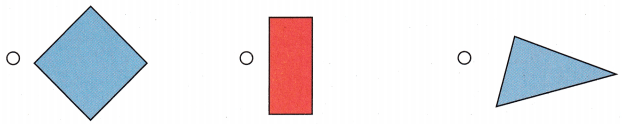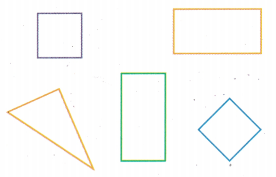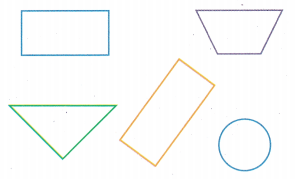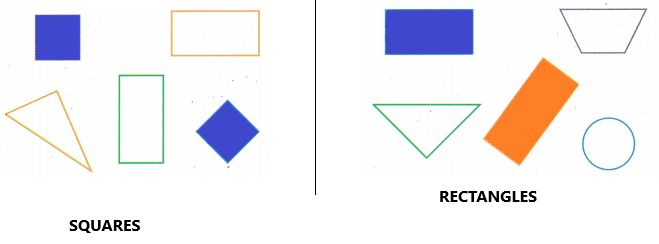# Texas Go Math Grade 1 Lesson 14.1 Answer Key Classify and Sort Two-Dimensional Shapes

Refer to our Texas Go Math Grade 1 Answer Key Pdf to score good marks in the exams. Test yourself by practicing the problems from Texas Go Math Grade 1 Lesson 14.1 Answer Key Classify and Sort Two-Dimensional Shapes.

## Texas Go Math Grade 1 Lesson 14.1 Answer Key Classify and Sort Two-Dimensional Shapes

Explore

Draw to sort the shapes. Write the sorting rule.We can sort two-dimensional shapes into groups.
A sorting rule is a rule that tells us how to sort objects.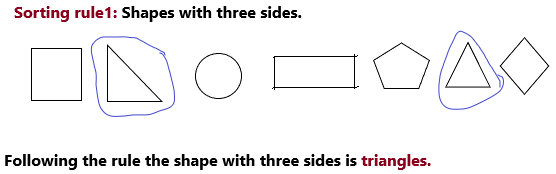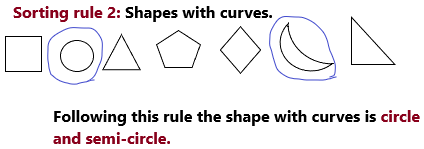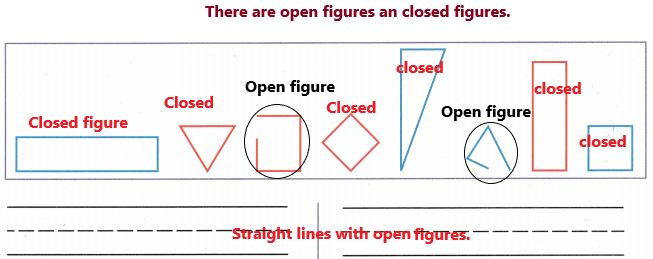FOR THE TEACHER • Read the following aloud. Devon wants to sort these shapes to show a group of triangles and a group of rectangles. Draw and write to show how Devon sorts the shapes.
Answer: Here the question was asked that we need to sort a group of triangles and rectangles. We will represent by diagrammatically.
First, we can sort two-dimensional shapes by comparing:
1. How many sides do they have.
2. How many vertices(corners) do they have.
3. If they have curves or not.
4. The length of their sides.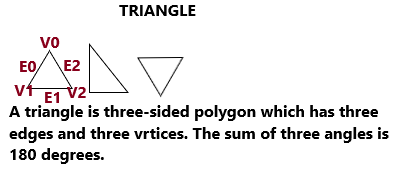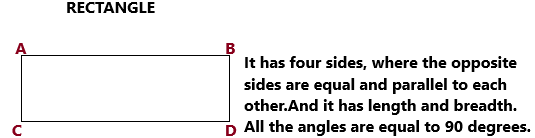Math Talk
Mathematical Processes

Is there a shaper that did not go in your groups? Explain.
Explanation:
No, there are no shapes that did not go in your group, because here we are dealing with two-dimensional shapes and we have triangle, square, circle, rectangle and so on. from the above questions so all of them are grouped. Here are some ways to sort two-dimensional shapes.

Model and Draw

Here are some ways to classify and sort two-dimensional shapes.

A square is a special kind of a rectangle.Answer: Closed shape with no sides and no corners are the circles.

closed shapes with ____3____ sides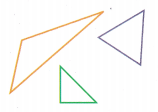triangles
Explanation:closed shapes with ____4____ vertices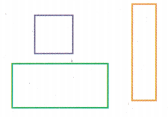rectangles
Explanation: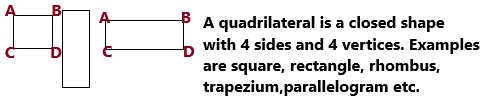Share and Show

THINK: Vertices (corners) are where the sides meet.

Question 1.
4 vertices (corners)Answer: Rectangle, square, trapezium are having the 4 vertices.
Explanation: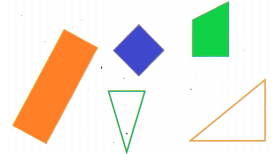The coloured ones are meeting the two sides and also have 4 vertices.

Question 2.
not curved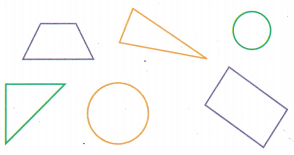Answer: There are 4 shapes that are not curved. They are parallelogram, triangles, trapezium.
Explanation:The coloured ones are not curved. These shapes are having sides and corners.

Question 3.
triangles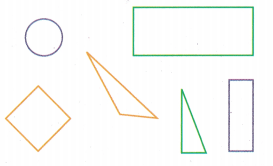Answer: In the given diagram, there are two triangles.
Explanation: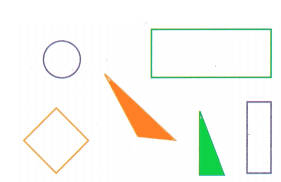The coloured ones are the triangles.

Question 4.
more than 3 sides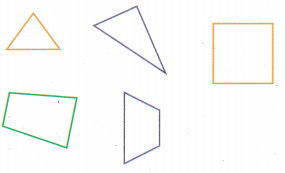Answer: There are 3 shapes that are more than 3 sides.
Explanation: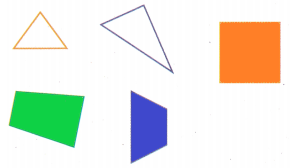The coloured ones are square, rectangle, trapezium. These shapes are having more than 3 sides.

Problem Solving

Circle the special rectangles in Exercise 6.

Question 5.
Color the shapes that are circles.Answer: In the below diagram, the shapes of circles are coloured.Question 6.
Color the squares red. Color other rectangles blue.Answer: In the below diagram, the shapes of circles are coloured.H.O.T. Draw 2 different two-dimensional shapes that follow both parts of the sorting rule.
Answer:Explanation:
– Both are having the same characteristics that follow the sorting rule are having 4 vertices and 4 sides. Moreover, length and breadth are also as follows.Question 7.
3 sides and 3 vertices (corners)
Explanation: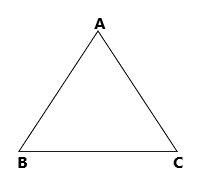Triangle is having 3 sides and 3 vertices.

Question 8.
2 sides are long and 2 sides are short
Explanation:Opposite sides are equal.

Question 9.
H.O.T. Multi-Step Write sorting rules to show two different ways to sort some of these shapes. Then color the shapes that follow one of your rules.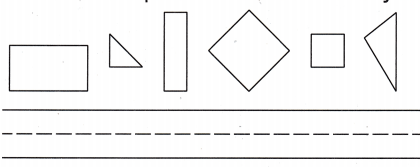Explanation:
1. I have coloured shapes having with 4 sides.
2. Others that are not coloured are having with 3 sides.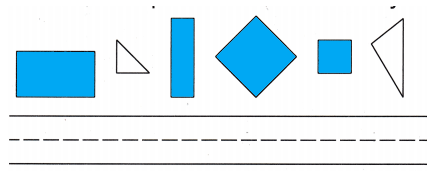Question 10.
Analyze Which shape is a rectangle?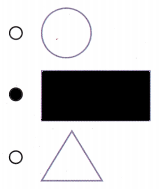Question 11.
Multi-Step Circle the triangles. Then circle the sorting rule you used.Answer: The representation has shown in the below diagram.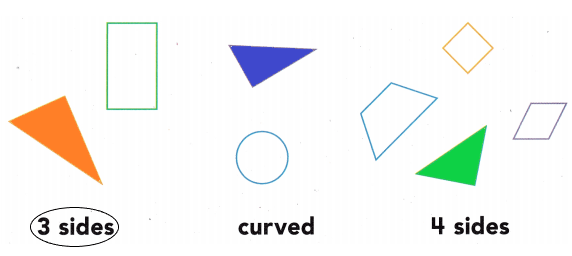Question 12.
Texas Test Prep Which shape would not be sorted into this group?Answer: The middle one cannot be sorted.
Explanation: The rhombus cannot be sorted because it has 4 sides and 4 corners but the other two was the triangle that are having 3 sides.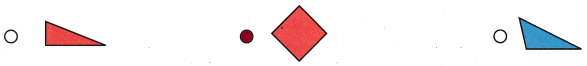TAKE HOME ACTIVITY • Gather some household objects such as photos. coins, and napkins. Ask your child to sort them by shape.
Answer: Make your child do the activity. Ask them to do square, rectangle, triangle, rhombus, trapezium, parallelogram, etc.

### Texas Go Math Grade 1 Lesson 14.1 Homework and Practice Answer Key

Question 1.
more than 3 sidesExplanation:
Here, the question was asking more than 3 sides. So it was a rectangle and square that are having 4 sides.Question 2.
circles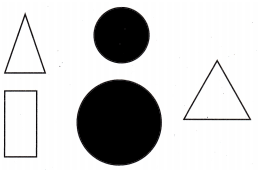Problem Solving

Draw 2 different two-dimensional shapes that follow both parts of the sorting rule.

Question 3.
4 sides are the same length
Explanation:Square: All sides are equal.

• All four interior angles are equal to 90°
• All four sides of the square are congruent or equal to each other
• The opposite sides of the square are parallel to each other
• The diagonals of the square bisect each other at 90°
• The two diagonals of the square are equal to each other

Question 4.
Multi-Step Write sorting rules to show two different ways to sort some of these shapes.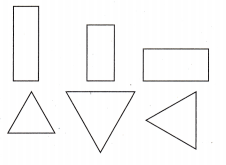Explanation:Rectangle sorting rules:

The fundamental properties of rectangles are:

• A rectangle is a quadrilateral
• The opposite sides are parallel and equal to each other
• Each interior angle is equal to 90 degrees
• The sum of all the interior angles is equal to 360 degrees
• The diagonals bisect each other
• Both the diagonals have the same length

Triangle sorting rules:

• The sum of all the angles of a triangle (of all types) is equal to 180°.
• The sum of the length of the two sides of a triangle is greater than the length of the third side.
• In the same way, the difference between the two sides of a triangle is less than the length of the third side.
• The side opposite the greater angle is the longest side of all the three sides of a triangle.

Lesson Check

Question 5.
Which shape is not curved?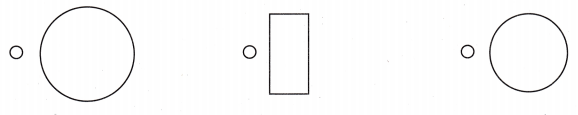Explanation: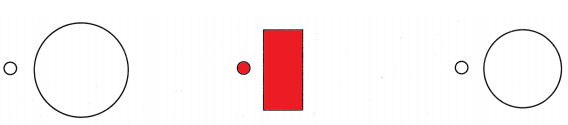Question 6.
Which shape is a special rectangle?